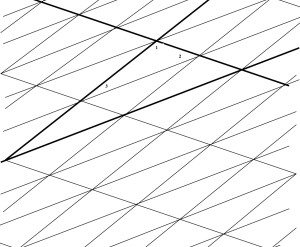What do you see in this diagram?
Look at the triangle with angles marked 1, 2 and 3. Call this T.
Label angles equal to these angles. Why are they equal?
Look at the larger heavily outlined triangle. How many copies of triangle T can you see in this triangle? What else do you notice about this triangle?

Click here for a copy of the diagram, here for a worksheet and here for Notes for Teachers.

Tagged with: